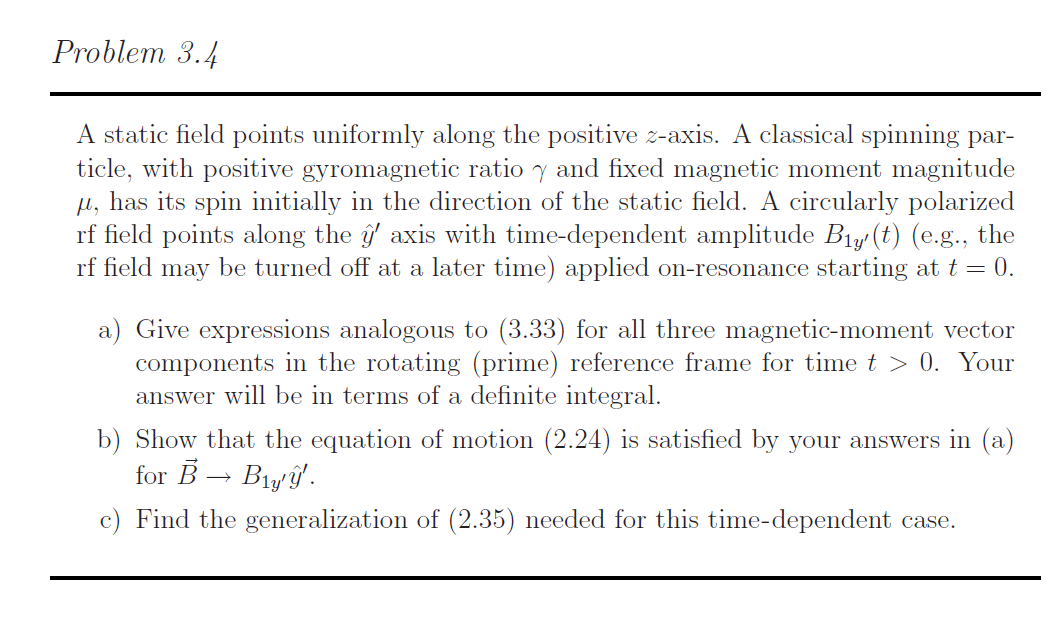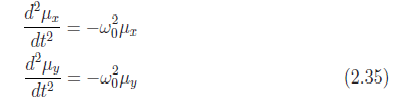# Problem 3.4A static field points uniformly along the positive z-axis. A classical spinning par-ticle, with positive gyromagnetic ratio y and fixed magnetic moment magnitude, has its spin initially in the direction of the static field. A circularly polarizedrf field points along the axis with time-dependent amplitude Big/(t) (e.g., therf field may be turned off at a later time) applied on-resonance starting at t 0.a) Give expressions analogous to (3.33) for all three magnetic-moment vectorcomponents in the rotating (prime) reference frame for time t > 0. Youranswer will be in terms of a definite integralb) Show that the equation of motion (2.24) is satisfied by your answers in (a)for BBlyc) Find the generalization of (2.35) needed for this time-dependent case. dt2(2.35)dt2

Question
173 views

textbook: Magnetic resonance imaging, physical principles and sequence design.

Problem 3.4

eq 3.33 : μx′(t) = μx′(0)
μy′(t) = μy′(0) cos φ1(t) + μz′(0) sin φ1(t)
μz′(t) = −μy′(0) sin φ1(t) + μz′(0) cos φ1(t)

eq 2.24: d~μ/dt
= γ~μ × ~Bhelp_outlineImage TranscriptioncloseProblem 3.4 A static field points uniformly along the positive z-axis. A classical spinning par- ticle, with positive gyromagnetic ratio y and fixed magnetic moment magnitude , has its spin initially in the direction of the static field. A circularly polarized rf field points along the axis with time-dependent amplitude Big/(t) (e.g., the rf field may be turned off at a later time) applied on-resonance starting at t 0. a) Give expressions analogous to (3.33) for all three magnetic-moment vector components in the rotating (prime) reference frame for time t > 0. Your answer will be in terms of a definite integral b) Show that the equation of motion (2.24) is satisfied by your answers in (a) for BBly c) Find the generalization of (2.35) needed for this time-dependent case. fullscreenhelp_outlineImage Transcriptionclosedt2 (2.35) dt2 fullscreen
check_circle

### Want to see the full answer?

See Solution

#### Want to see this answer and more?

Solutions are written by subject experts who are available 24/7. Questions are typically answered within 1 hour.*

See Solution
*Response times may vary by subject and question.
Tagged in

### Electrical Engineering